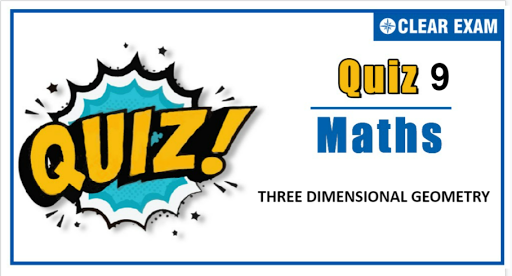## Three Dimensional Geometry Quiz

Important topics for Maths has been designed in such a way that it offers very practical and application-based learning to further make it easier for students to understand every concept or topic by correlating it with the day-to-day experiences.

Q1. The lines: (x-2)/1=(y-3)/1=(z-4)/(-k) and (x-1)/k=(y-4)/2=(z-5)/1 are coplanar if:
•  k=1 or -1
•  k=0 or -3
•  k=3 or -3
•  k=0 or -1
•  Parallel lines
•  Coplanar lines
•  Coincident lines
•  Concurrent lines
Solution
(d) P_1=P_2=0,P_2=P_3=0 and P_3=P_1=0 are lines of intersection of the three planes P_1,P_2 and P_3. As n ⃗_1,n ⃗_2 and n ⃗_3 are non-coplanar, planes P_1,P_2 and P_3 will intersect at unique point. So the given liens will pass through a fixed point

Q3.  The pair of lines whose direction cosines are given by the equations 3l+m+5n=0 and 6mn-2nl+5lm=0, are
•  Parallel
•  Perpendicular
•  Inclined at cos^(-1)⁡(1/6)
•  None of these
Solution
(c) 3l+m+5n=0 (i) 6mn-2nl+5ml=0 (ii) Substituting the value of n from Eq. (i) in Eq. (ii), we get 6l^2+9lm-6m^2=0 ⇒ 6(l/m)^2+9(l/m)-6=0 ∴l_1/m_1 =1/2 and l_2/m_2 =-2 From Eq. (i), we get l_1/n_1 =-1 and l_2/n_2 =-2 ∴l_1/1=m_1/2=n_1/(-1)=√((l_1^2+m_1^2+n_1^2)/(1+4+1))=1/√6 and l_2/2=m_2/(-1)=n_2/(-1)=√(l_2^2+m_2^2+n_2^2 )/√(4+1+1)=1/√6 If θ be the angle between the lines, then cos⁡θ=(1/√6)(2/√6)+(2/√6)(-1/√6)+(-1/√6)(-1/√6)=1/6 ∴ θ=cos^(-1)⁡(1/6)

Q4. The centre of the circle given by: r ∙(i ̂+2j ̂+2k ̂ )=15 and |r -(j ̂+2k ̂ )|=4 is
•  (0,1,2)
•  (1,3,4)
•  (-1,3,4)
•  None of these
Solution
(b) The equation of the line through the centre j ̂+2k ̂ and normal to the given plane is r =j ̂+2k ̂+λ(i ̂+2j ̂+2k ̂) (i) This meets the plane for which [j ̂+2k ̂+λ(i ̂+2j ̂+2k ̂ )]∙(i ̂+2j ̂+2k ̂)=15 ⇒6+9λ=15 ⇒ λ=1 Putting in (i), we get r =j ̂+2k ̂+(i ̂+2j ̂+2k ̂ )=i ̂+3j ̂+4k ̂ Hence, centre is (1, 3, 4)

Q6. The equation of the plane through the intersection of the planes x+2y+3z-4=0 and 4x+3y+2z+1=0 and passing through the origin is
•  17x+14y+11z=0
•  7x+4y+z=0
• x+14y+11z=0
•  17x+y+z=0
Solution
(a) Any plane through the given planes is x+2y+3z-4+λ(4x+3y+2z+1)=0 It passes through (0,0,0). Therefore, -4+λ=0 ∴ λ=4 Therefore, the required plane is x+2y+3z+4(4x+3y+2z)=0 or 17x+14y+11z=0

Q7. The equation of the plane through the line of intersection of the planes ax+by+cz+d=0 and a^' x+b^' y+c^' z+d^'=0 and parallel to the line y=0 and z=0 is
•  None of these
Q8.A variable planex/a+y/b+z/c=1 at a unit distance from origin cuts the coordinate axes at A,B and C.Centriod (x,y,z)satisfies the equation1/x^2 +1/y^2 +1/z^2 =k.The value of k is
•  9
•  3
•  1/9
•  1/3
Solution
a) As x/a+y/b+z/c=1 cuts the coordinate axes at A(a,0 ,0),B(0,b,0),C(0,0,c) Since, distance from origin =1 ⟹1/√(1/a^2 +1/b^2 +1/c^2 )=1 ⟹ 1/a^2 +1/b^2 +1/c^2 =1 …..(i) ∴ Centroid P(x,y,z)=((a+0+0)/3,(0+b+0)/3,(0+0+c)/3) ⟹x=a/3,y=b/3,z=c/3 ..(ii) From Eqs. (i) and (ii), 1/〖9x〗^2 +1/(9y^2 )+1/(9z^2 )=1 ⟹ 1/x^2 +1/y^2 +1/z^2 =9=k (given) ⟹k=9

Q9. The plane, which passes through the point (3, 2, 0) and the line (x-3)/1=(y-6)/5=(z–4)/4 is:
•  x-y+z=1
•  x+y+z=5
•  x+2y-z=1
•  2x-y+z=5
Q10. The point of intersection of the line passing through (0,0, 1) and intersecting the lines x+2y+z=1,-x+y-2z=2 and x+y=2,x+z=2 with xy plane is
•  (5/3,-1/3,0)
•  (1,1,0)
•  (2/3,-1/3,0)
• (-5/3,1/3,0)
Solution
(a) Equation of line x+2y+z-1+λ(-x+y-2z-2)=0 (i) x+y-2+μ(x+z-2)=0 (ii) (0, 0, 1) lies on it ⇒λ=0,μ=-2 For point of intersection, z=0 and solve (i) and (ii)#### Written by: AUTHORNAME

AUTHORDESCRIPTION## Want to know more

Please fill in the details below:

## Latest NEET Articles\$type=three\$c=3\$author=hide\$comment=hide\$rm=hide\$date=hide\$snippet=hide

Name

ltr
item
BEST NEET COACHING CENTER | BEST IIT JEE COACHING INSTITUTE | BEST NEET & IIT JEE COACHING: Three-Dimensional-Geometry-Quiz-9
Three-Dimensional-Geometry-Quiz-9